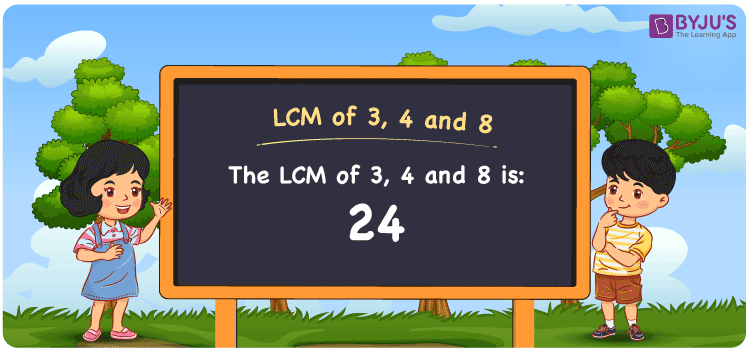# LCM of 3 4 and 8

LCM of 3, 4 and 8 is 24. LCM stands for Least Common Multiple or the Lowest Common Multiple also known as LCD Least Common Divisor is the positive integer that is a common multiple to the given set of numbers. Here the given set of numbers are 3, 4 and 8. The smallest common multiple for all these numbers among many is 24, and hence it is the LCM. You can refer to LCM with Examples to understand better.

## What is LCM of 3, 4 and 8

The Least Common Multiple or Lowest Common Multiple of 3, 4 and 8 is 24.## How to Find LCM of 3, 4 and 8?

LCM of 3, 4 and 8 can be determined using three methods:

• Prime Factorisation
• Division method
• Listing the multiples

### LCM of 3, 4 and 8 Using Prime Factorisation Method

In the Prime Factorisation method, the numbers 3, 4 and 8 can be expressed as;

3 = 1 × 3

4 = 2 × 2

8 = 2 × 2 × 2

The product of common factors and uncommon factors of 3, 4 and 8 forms the LCM.

LCM(3, 4 and 8) = 2 × 2 × 2 × 3 = 24

### LCM of 3, 4 and 8 Using Division Method

In the Division Method, the given set of numbers 3, 4 and 8 are divided by prime divisors. The product of prime divisors forms the LCM.

 2 3 4 8 2 3 2 4 2 3 1 2 3 3 1 1 × 1 1 1

LCM(3, 4 and 8) = 2 × 2 × 2 × 3 = 24

### LCM of 3, 4 and 8 Using Listing the Multiples

By listing all the multiples of 3, 4 and 8, we can identify the LCM. Below is the list of multiples for 3, 4 and 8.

 Multiples of 3 Multiples of 4 Multiples of 8 3 4 8 6 8 16 9 12 24 12 16 32 15 20 40 18 24 48 21 28 56 24 32 64

LCM(3, 4 and 8) = 24

## Video Lesson on Applications of LCM## Solved Examples

What is the smallest number that is divisible by 3, 4 and 8?

Answer: 24 is the smallest number that is divisible by 3, 4 and 8.

What is the LCM of 3 and 24?

Answer: LCM of 3 and 24 is 24.

## Frequently Asked Questions on LCM of 3, 4 and 8

### What is the LCM of 3, 4 and 8?

The LCM of 3, 4 and 8 is 24.

### What is the difference between the LCM 3, 4 and 8 and HCF of 3, 4 and 8?

The LCM of 3, 4 and 8 is 24 and HCF of 3, 4 and 8 is 1.

### Is the LCM and LCD both the same for 3, 4 and 8?

Yes. The LCM and Lowest Common Divisor are both the same for 3, 4 and 8  which is 24.

### What are the first 3 common multiples of 3, 4 and 8?

The first three common multiples of 3, 4 and 8 are 24, 48, and 72.

### Is the LCM of 3 and 8 the same as the LCM of 4 and 8?

No. LCM of 3 and 8 is 24, whereas the LCM of 4 and 8 is 8. They are not the same.